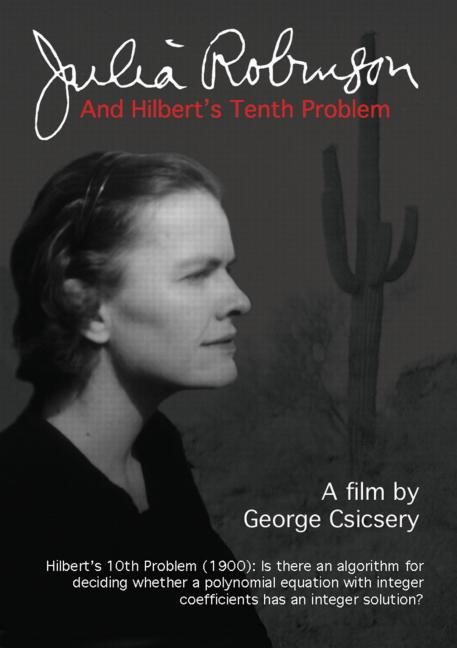# Enumerating Diophantine equations

I mentioned this problem in passing in my last post, but it’s really not trivial. Here’s the method exposed in Matiyasevich’s Hilbert’s Tenth Problem. The method originates from Julia Robinson, in Diophantine Decision Problems (in Studies in Number Theory).

Here are the rules she offers to generate all integer polynomials Pm and all positive integer polynomials Tn:

• T4n = n
• T4n+1 = xn
• T4n+2 = TCantorLeft(n) + TCantorRight(n)
• T4n+3 = TCantorLeft(n) * TCantorRight(n)
• Pm = TCantorLeft(m) - TCantorRight(m)

As you can see, Tn will includes all possible constants and unknowns, as well as any sums or products of other Tn elements. Then Pm will be the difference of any two Tn elements.

An example: P7,297,614,550 = 2.x02 - (1 + x12)

One thing to notice is that Tn will generate duplicates. For instance, T4n+2 = T4m+2 when CantorLeft(n) = CantorRight(m) and CantorRight(n) = CantorLeft(m). I don’t know if there is a numbering that avoids such duplication.